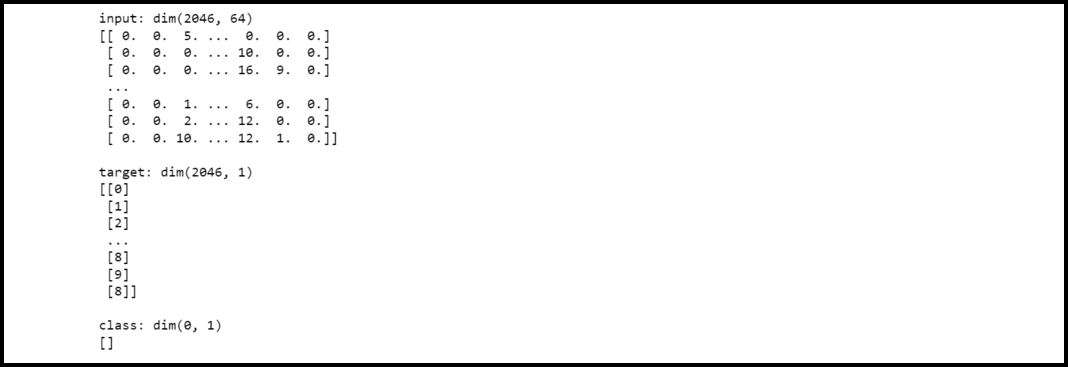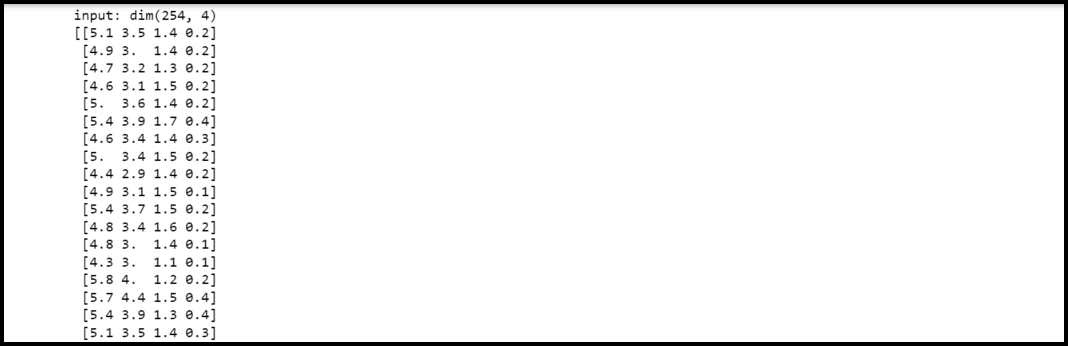GeeksforGeeks App
Open AppBrowser
Continue

# How to import datasets using sklearn in PyBrain

In this article, we will discuss how to import datasets using sklearn in PyBrain

Dataset: A Dataset is defined as the set of data that is can be used to test, validate, and train on networks. On comparing it with arrays, a dataset is considered more flexible and easy to use. A dataset resembles a 2-d array. Datasets are used in executing machine learning tasks.

Libraries need to be installed in our system are:

• sklearn
• pybrain

Syntax to install these libraries :

`pip install sklearn pybrain`

Example 1:

In this example, firstly we have imported packages datasets from sklearn library and ClassificationDataset from pybrain.datasets. Then we have loaded the digits dataset. In the next statement, we are defining feature variables and target variables.  Then we are creating a classification dataset model by defining 64 inputs, 1 output, and 15 classes. Then, we have appended data to the created dataset.

## Python3

 `# Importing libraries``from` `sklearn ``import` `datasets``from` `pybrain.datasets ``import` `ClassificationDataSet`` ` `# Loading digits``loaded_digits ``=` `datasets.load_digits()`` ` `# Set data items``x_data, y_data ``=` `loaded_digits.data, loaded_digits.target`` ` `# Classification dataset``dataset ``=` `ClassificationDataSet(``64``, ``1``, nb_classes``=``15``)`` ` `# Iterate over the length of X``for` `i ``in` `range``(``len``(x_data)):``    ``dataset.addSample(x_data[i], y_data[i])`` ` `# Print the dataset``print``(dataset)`

Output:Example 2:

In this example, firstly we have imported packages datasets from sklearn library and ClassificationDataset from pybrain.datasets. Then we have loaded the iris dataset. In the next statement, we are defining feature variables and target variables.  Then we are creating a classification dataset model by defining 4 inputs, 1 output, and 2 classes. Then, we have appended data to the created dataset.

## Python3

 `# Importing libraries``from` `sklearn ``import` `datasets``from` `pybrain.datasets ``import` `ClassificationDataSet`` ` `# Loading iris``loaded_digits ``=` `datasets.load_iris()`` ` `# Setting data fields``x_data, y_data ``=` `loaded_digits.data, loaded_digits.target`` ` `# Creating a ClassificationDataset``dataset ``=` `ClassificationDataSet(``4``, ``1``, nb_classes``=``2``)`` ` `# Iterating over the length of x_data``for` `i ``in` `range``(``len``(x_data)):``    ``dataset.addSample(x_data[i], y_data[i])`` ` `# Print the dataset``print``(dataset)`

Output:My Personal Notes arrow_drop_up